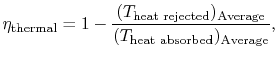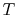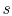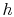Thermodynamics and Propulsion

# 8.8 Some Overall Comments on Thermodynamic Cycles

1. There are many different power and propulsion cycles, and we have only looked at a few of these. Many other cycles have been devised in the search for ways to increase efficiency and power in practical devices.
2. We can view a given cycle in terms of elementary Carnot cycles, as sketched in Figure 6.5. This shows that the efficiency of any other cycle operating between two given temperatures will be less than that of a Carnot cycle.
3. If we view the thermal efficiency as(derived in Section 8.5), this means that we should accept heat at a high temperature and reject it at a low temperature for high efficiency. This objective must be tempered by considerations of practical application.
4. The cycle diagrams in-and-coordinates will only be similar if the working medium is an ideal gas. For other media (for example, a two-phase mixture) they will look different.
5. Combined cycles make use of the rejected heat from a topping'' cycle as heat source for a bottoming'' cycle. The overall efficiency is higher than the efficiency of either cycle.

UnifiedTP